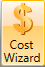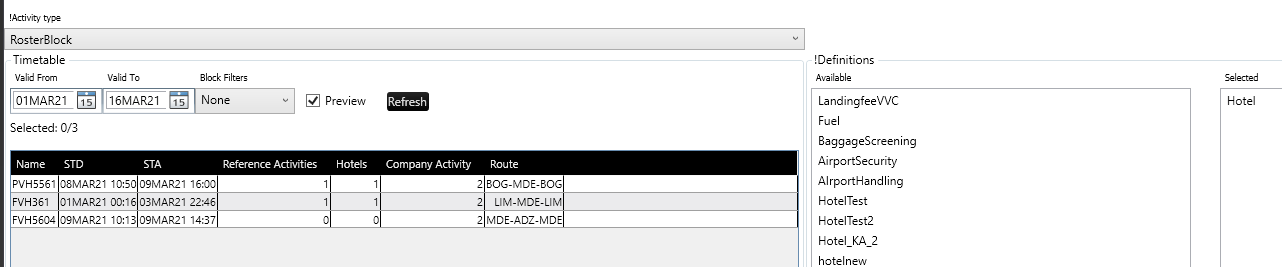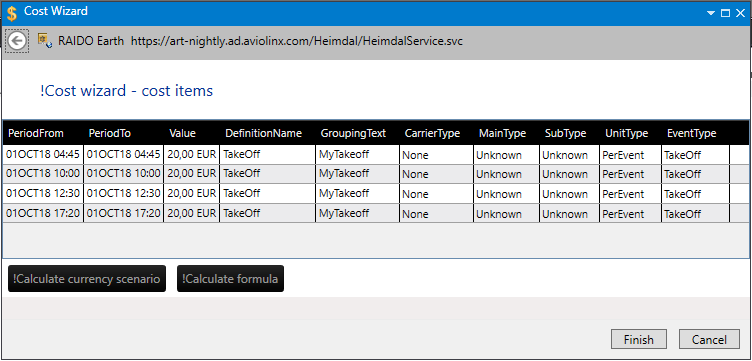# Cost WizardCost wizard currently contains a couple of pages.
1st page page is used to select wanted flights and definitions that shall be calculated on those flights.
The page is divided into a few parts:
Timetable - allows user to select flights for costs calculation (using ctrl or shift key one can select more than 1 flight/leg) with the support of following controls:
 Activity type Select if you want to work with Rosterblock (pairings) or Company activity (legs) valid from - valid to (period of flights that are to be shown for selection in the table below) activity/Activity filter filter the legs within the selected period by the means of already defined activity filters in the current environment preview checkbox load the legs into the table refresh button refresh the table with the legs (i.e. when filter or period has been changed or one would like the legs data to be refreshed) Definitions Select cost definitions that shall be used during cost calculation. If formula requires i.e. Fuel cost definition and it is not in the right-hand side (Selected) column then an error will be shown in the status bar of the dialog. Please mind that if i.e. formula requires Fuel cost definition and there was selected Fuel2 or FuelScenarioX in the Definitions then an error will be visible in the status bar of the dialog because selected definitions does not match (1 to 1) with the definition used in selected/given formula2nd page shows calculated cost items - so the flights are transformed into events and those events based on the selected definitions have their values calculated (the data are not aggregated in this step)

If the user would like to process the data additionally one can select all rows (i.e. with ctrl+a) and copy them into clipboard (ctrl+c) - then one can paste it into any spreadsheet to make i.e. pivot table.

There are 2 additional options (bottom left buttons) available for the user:

Calculate currency scenario - allows user to change the currency based on the selected (previously defined via Exchange rate table dialog) currency scenario into wanted 1 currencyCalculate formula - allows user to aggregate cost items into a single value based on the given formula (see also Cost formula dialog)

Calculating formula page allows user to select:
 Currency scenarios Select exchange rate tables names that shall be used for costs calculation. Only scenarios that are in the right-hand side (Selected) column will be used by cost calculation. Result currency Select result currency in which the final cost value shall be calculated/shown in Formula choose formula from already defined ones (see also Cost Formula dialog)type in temporary cost formula that shall be used for the calculation - the same rules for the entered formula/expression applies as in the Cost Formula dialog (see also Cost Formula dialog)Did you find it helpful? Yes No

Send feedback